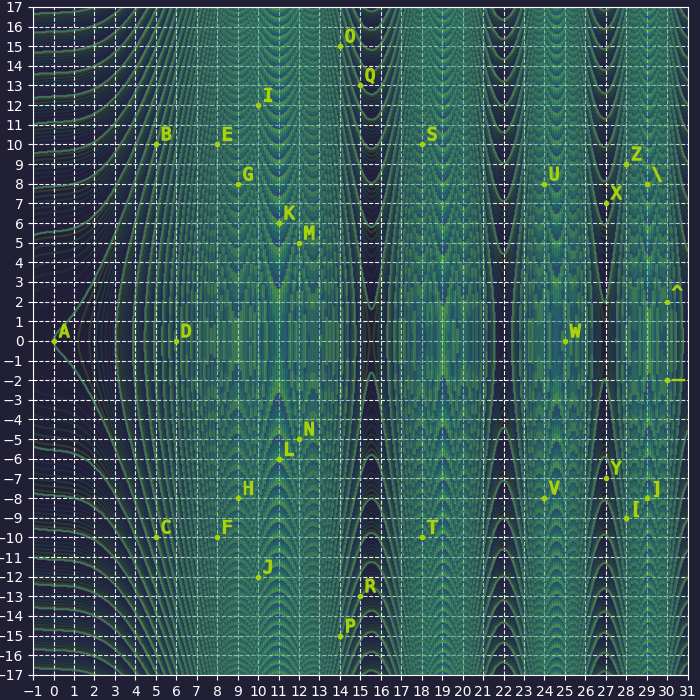Published on

# Ugra CTF Quals 2022 – noteasy03

Authors
•Name
Y4nhu1
@YanhuiJessica

## noteasy03 (crypto, 350)

Цезарь скривился,
Замкнулся в себе.
Преумножение.

Caesar curved,
closed in on himself.
Multiplication.

Attachments: ciphertext.txt
Flag Format: ugra_[A-Za-z0-9_]+According to curved, closed in on himself and the picture, we speculated that it should be some kind of curve. Meanwhile, all points can find corresponding points on the curve.

Considering the ciphertext and the flag format, we got the following mapping relationship and speculated that it’s a single table substitution according to Caesar.

r -> u
z -> g
u -> r
a -> a
] -> _


We excluded common single table substitution ciphers (all linear) and finally thought out Elliptic Curves.

Although the shape of the point distribution does not seem to match the Elliptic Curves in the real number field, the point set in the figure completely conforms to the characteristics of the Elliptic Curve in the FINITE field. Meanwhile, the inverse of a point on the Elliptic Curve can meet the mapping requirements of encryption and decryption.

I used the online Elliptic Curve visualization tool to find the approximate range of Elliptic Curve coefficients.

• Key feature: three points on the $x$ axis, one point on $(0, 0)$

And then, by combining the coordinates of the known points, we can determine the elliptic curve coefficients are $a=-5,b=0$

from Crypto.Util.number import *

ps = [(0, 0), (5, 10), (5, 21), (6, 0), (8, 10), (8, 21), (9, 8), (9, 23), (10, 12), (10, 19), (11, 6), (11, 25), (12, 5), (12, 26), (14, 15), (14, 16), (15, 13), (15, 18), (18, 10), (18, 21), (24, 8), (24, 23), (25, 0), (27, 7), (27, 24), (28, 9), (28, 22), (29, 8), (29, 23), (30, 2), (30, 29)]

for a in range(-5, 0):
for b in range(-4, 5):
# According to the coordinates of each point, the finite field size is 31
if 4 * a**3 + 27 * b**2:
E = EllipticCurve(Zmod(31), [a, b])
else: continue
f = 1
for p in ps:
try: E(p, p)
except:
f = 0
break
if f: print(a, b)


I guessed that the mapping of points is a point multiplication in an Elliptic Curve with a coefficient of $3$ inferred from Caesar and Multiplication. But the decrypted result is a bunch of garbled characters.

A shift of 3 is for the original Caesar cipher. So, I try to change the coefficient and successfully get the FLAG when the coefficient is $11$ XD

from Crypto.Util.number import *

cipher = 'rzua]o^]tahf]ie]kiho^z]niru]ha^ogn]doak]i[]g[uff]iop^atpe[paz[[tapzetd'

E = EllipticCurve(Zmod(31), [-5, 0])
d = {E(0, 0): 'a', E(5, 10): 'b', E(5, 21): 'c', E(6, 0): 'd', E(8, 10): 'e', E(8, 21): 'f', E(9, 8): 'g', E(9, 23): 'h', E(10, 12): 'i', E(10, 19): 'j', E(11, 6): 'k', E(11, 25): 'l', E(12, 5): 'm', E(12, 26): 'n', E(14, 15): 'o', E(14, 16): 'p', E(15, 13): 'q', E(15, 18): 'r', E(18, 10): 's', E(18, 21): 't', E(24, 8): 'u', E(24, 23): 'v', E(25, 0): 'w', E(27, 7): 'x', E(27, 24): 'y', E(28, 9): 'z', E(28, 22): '[', E(29, 8): '\\', E(29, 23): ']', E(30, 2): '^', E(30, 29): '_'}
res = dict()

for k, v in d.items():
res[v] = d[11 * k]

for c in cipher:
print(res[c], end='')

# ugra_in_case_of_losing_your_sanity_dial_oh_three_oijnacjfhjaghhcajgfcd